# Definite Integral - Calculus

Before going to learn about definite integrals, first, recollect the concept of integral. An integral assigns numbers to functions in mathematics to define displacement, area, volume, and other notions that arise by connecting infinitesimal data. The process of finding integrals is called integration. Definite integrals are used when the limits are defined to generate a unique value. Indefinite integrals are implemented when the boundaries of the integrand are not specified. In case, the lower limit and upper limit of the independent variable of a function are specified, its integration is described using definite integrals. Also, we have several integral formulas to deal with various definite integral problems in maths.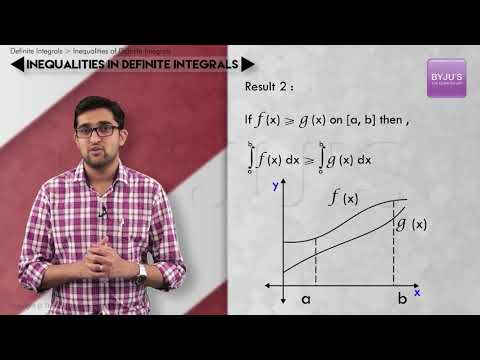## Definite Integral Definition

The definite integral of a real-valued function f(x) with respect to a real variable x on an interval [a, b] is expressed as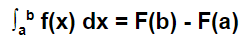Here,

∫ = Integration symbol

a = Lower limit

b = Upper limit

f(x) = Integrand

dx = Integrating agent

Thus, ∫ab f(x) dx is read as the definite integral of f(x) with respect to dx from a to b.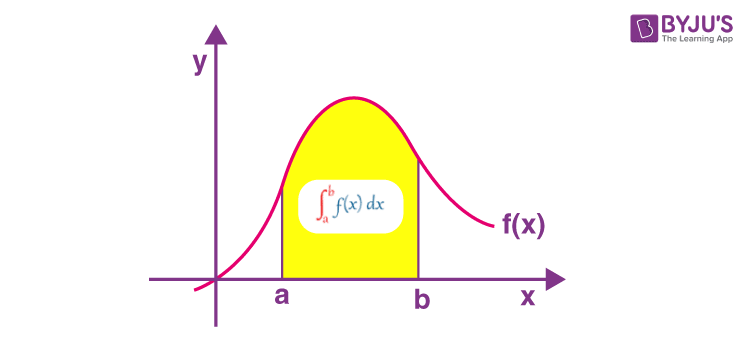## Definite Integral as Limit of Sum

The definite integral of any function can be expressed either as the limit of a sum or if there exists an antiderivative F for the interval [a, b], then the definite integral of the function is the difference of the values at points a and b. Let us discuss definite integrals as a limit of a sum. Consider a continuous function f in x defined in the closed interval [a, b]. Assuming that f(x) > 0, the following graph depicts f in x.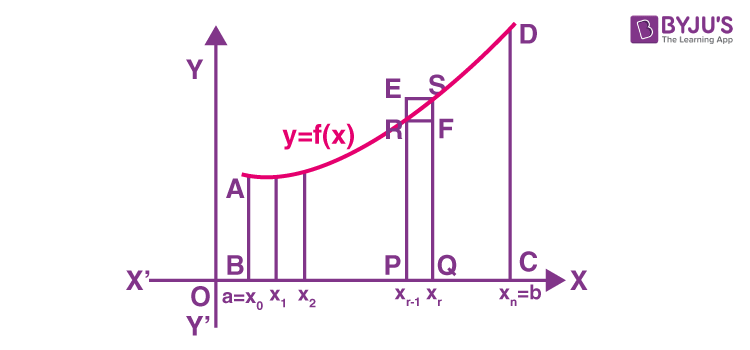The integral of f(x) is the area of the region bounded by the curve y = f(x). This area is represented by the region ABCD as shown in the above figure. This entire region lying between [a, b] is divided into n equal subintervals given by [x0, x1], [x1, x2], …… [xr-1, xr], [xn-1, xn].

Let us consider the width of each subinterval as h such that h → 0, x0 = a, x1 = a + h, x2 = a + 2h,…..,xr = a + rh, xn = b = a + nh

and n = (b – a)/h

Also, n→∞ in the above representation.

Now, from the above figure, we write the areas of particular regions and intervals as:

Area of rectangle PQFR < area of the region PQSRP < area of rectangle PQSE ….(1)

Since. h→ 0, therefore xr–  xr-1→ 0. The following sums can be established as;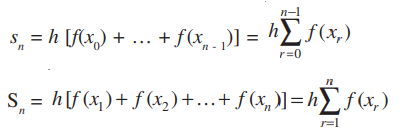From the first inequality, considering any arbitrary subinterval [xr-1, xr] where r = 1, 2, 3….n, it can be said that sn< area of the region ABCD <Sn

Since, n→∞, the rectangular strips are very narrow, it can be assumed that the limiting values of sn and Sn are equal, and the common limiting value gives us the area under the curve, i.e.,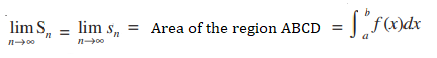From this, it can be said that this area is also the limiting value of an area lying between the rectangles below and above the curve. Therefore,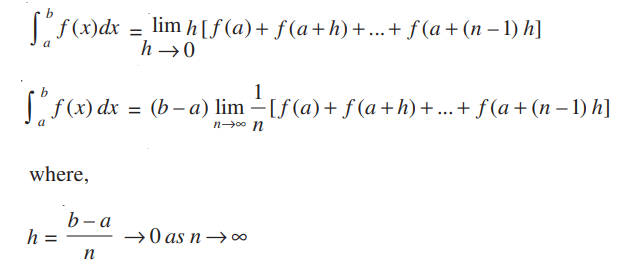This is known as the definition of definite integral as the limit of sum.

## Definite Integral Properties

Below is the list of some essential properties of definite integrals. These will help evaluate the definite integrals more efficiently.

• af(x) dx = ∫af(t) d(t)
• af(x) dx = – ∫bf(x) dx
• af(x) dx = 0
• af(x) dx = ∫ac f(x) dx + ∫cb f(x) dx
• af(x) dx = ∫ab f(a + b – x) dx
• 0f(x) dx =  f(a – x) dx

Steps for calculating ∫ab f(x) dx

Step 1: Find the indefinite integral ∫f(x) dx. Let this be F(x). There is no need to keep

integration constant C. This is because if we consider F(x) + C instead of F(x), we get

ab f(x) dx = [F(x) + C]ab = [F(b) + C] – [F(a) + C] = F(b) + C – F(a) – C = F(b) – F(a)

Thus, the arbitrary constant will not appear in evaluating the value of the definite integral.

Step 2: Calculate the value of F(b) – F(a) = [F(x)]ab

Hence, the value of ab f(x) dx = F(b) – F(a)

### Definite Integral by Parts

Below are the formulas to find the definite integral of a function by splitting it into parts.

• 02a f (x) dx = ∫0a f (x) dx + ∫0f (2a – x) dx
• 02a f (x) dx = 2 ∫0f (x) dx … if f(2a – x) = f (x).
• 02a f (x) dx = 0 … if f (2a – x) = – f(x)
• -aa f(x) dx = 2 ∫0f(x) dx … if f(- x) = f(x) or it is an even function
• -aa f(x) dx = 0 … if f(- x) = – f(x) or it is an odd function

### Definite Integral Examples

Example 1:

Evaluate the value of ∫23 x2 dx.

Solution:

Let I = ∫2x2 dx

Now, ∫x2 dx = (x3)/3

Now, I =  ∫2x2 dx = [(x3)/3]23

= (33)/3 – (23)/3

= (27/3) – (8/3)

= (27 – 8)/3

= 19/3

Therefore, ∫23 x2 dx = 19/3

Example 2:

Calculate: ∫0π/4 sin 2x dx

Solution:

Let I = ∫0 π/4 sin 2x dx

Now, ∫ sin 2x dx = -(½) cos 2x

I = ∫0 π/4 sin 2x dx

= [-(½) cos 2x]0π/4

= -(½) cos 2(π/4) – {-(½) cos 2(0)}

= -(½) cos π/2 + (½) cos 0

= -(½) (0) + (½)

= 1/2

Therefore, ∫0 π/4 sin 2x dx = 1/2

### Definite Integration – Short Notes for Quick Revision## Frequently Asked Questions on Definite Integral

Q1

### What is a definite integral?

The definite integral has a unique value. A definite integral is denoted by ∫ab f(x) dx, where a is called the lower limit of the integral and b is called the upper limit of the integral.
Q2

### What is the formula for definite integral?

The formula for calculating the definite integral of a function f(x) in the interval [a, b] is given by,
ab f(x) dx = F(b) – F(a)
Q3

### What is a definite integral used for?

We can use definite integrals to find the area under, over, or between curves in calculus. If a function is strictly positive, the area between the curve of the function and the x-axis is equal to the definite integral of the function in the given interval. In the case of a negative function, the area will be -1 times the definite integral.
Q4

### Can a definite integral be negative?

Yes, the value of a definite integral can be negative, positive or zero.
Q5

### Do definite integrals have C?

No, definite integrals do not have C. As it is not required to add an arbitrary constant, i.e. C in case of definite integrals.